Next: Layout of parallel Up: Parallel Algorithm Analysis Previous: Parallel Communications Model

## CM-5 Communications Model

As described in Subsection 2.1, we use (1) as the general model for CM-5 communications. As stated above, a common communications pattern on parallel machines is a block permutation, where given blocks of contiguous data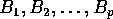, and a permutation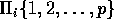, and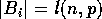, block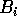has to be moved from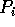to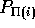. If each block has length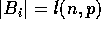, we claim that the CM-5 time complexity of this operation is the following: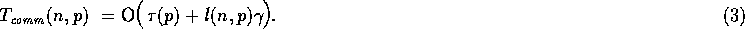Each node in the CM-5 connects to the fat-tree via a 20 Mb/sec network interface link . The fat-tree network provides sufficient bandwidth to allow every node to perform sustained data transfers at a rate of 20 Mb/sec if all communications is contained in groups of four nodes (i.e. the nodes differ only in the last two bits of their logical address), 10 Mb/sec for transfers within groups of 16 nodes (i.e. the nodes differ in only the last four bits), and 5 Mb/sec for all other communication paths in the system .

A regular grid shift is an example of a block permutation pattern of data movement as shown in Subsection 2.2.2. For the corresponding regular block communications, the CM-5 can achieve bandwidths of 15 megabytes/sec per processor to put messages into and take messages out of the data network . The runtime system (RTS) of the CM-5 will choose a data section for a message of between 1 and 5 words in length. If we assume that the data section of a message,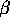, is four 4-byte words, and the header and trailer are an additional 4 bytes, then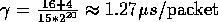.

To support these claims, we give the following empirical results. Using the message passing library of the CM-5, CMMD version 3.0 , each processing node swaps a packet with another node of fixed distance away. Figure 3 confirms that there is a linear relationship between message length and total transmission time on the CM-5. We find that when the regular block permutation consists of only local communications, i.e. messages are contained in each cluster of four leaf nodes,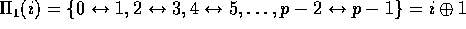, the lower bound on time is obtained. A least squares analysis of this data finds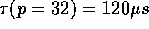and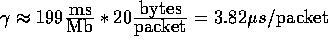. This is equivalent to a sustained transfer rate of 5.03 Mb/sec.

Other regular block permutations are shown in Figure 3 such as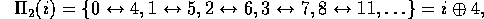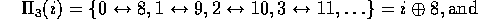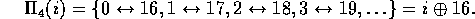Both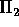and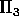use two levels of the fat-tree, while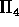needs three levels. Our results show that all non-local regular permutations routed through the fat-tree have similar time complexities, with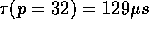and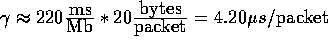. This corresponds to a sustained transfer rate of 4.55 Mb/sec.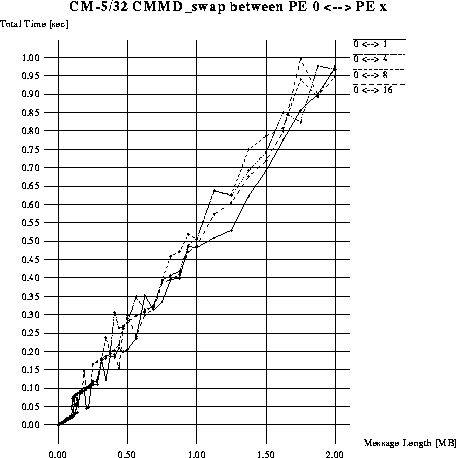Figure: Sending Time of a Regular Block PermutationNext: Layout of parallel Up: Parallel Algorithm Analysis Previous: Parallel Communications Model